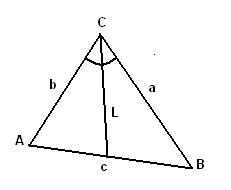homechevron_rightStudychevron_rightMathchevron_rightGeometry

# Angle bisector of a triangle

This online calculator computes the length of angle bisector given the lengths of triangle edgesThis content is licensed under Creative Commons Attribution/Share-Alike License 3.0 (Unported). That means you may freely redistribute or modify this content under the same license conditions and must attribute the original author by placing a hyperlink from your site to this work https://planetcalc.com/51/. Also, please do not modify any references to the original work (if any) contained in this content.This online calculator computes the length of the angle bisector given the lengths of triangle edges (see the picture). Triangle vertices are usually named A, B, and C. Triangle edges - a, b, c, where the letter denotes opposite vertex. That is the edge between A and B is named c, between A and C - b, between B and C - a.
The length of С's angle bisector shown on picture is:#### Angle bisector of a triangle

Digits after the decimal point: 2
Length of angle bisector

URL copied to clipboard

#### Similar calculatorsPLANETCALC, Angle bisector of a triangle